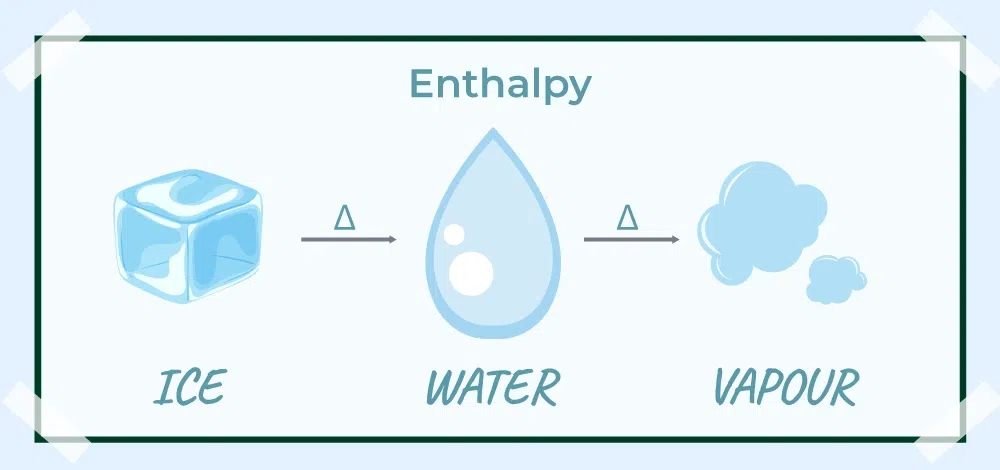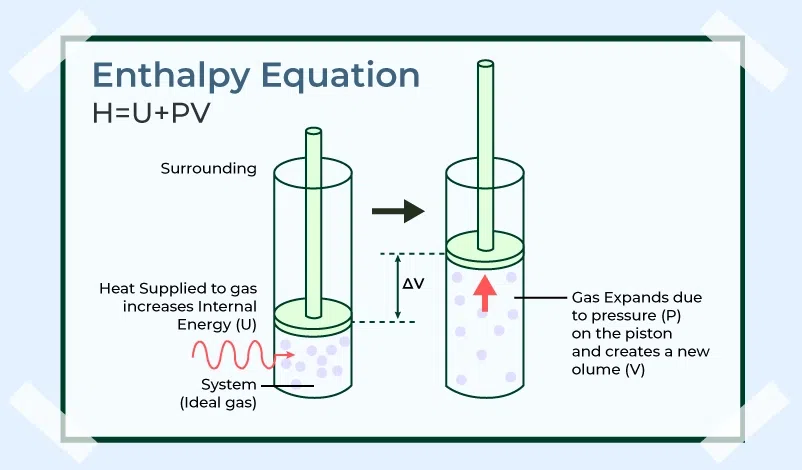Open In App
Related Articles
• CBSE Class 11 Chemistry Notes

# Enthalpy

Enthalpy is the measurement of heat or energy in the thermodynamic system, and it is the most fundamental concept in the branch of thermodynamics. Enthalpy plays an important role in many branches of science and engineering, including chemistry, physics, and the material sciences. There are some real-life applications of enthalpy, such as in power generation and chemical manufacturing. In this article, we will study the concept of enthalpy, its definition, formulas, and various relations between enthalpy and other quantities. There are various kinds of enthalpies related to the various processes such as enthalpy of atomization, enthalpy of formation, enthalpy of fusion, enthalpy of neutralization, enthalpy of hydration, etc.## What is Enthalpy?

Enthalpy is total energy o heat in the system which is equivalent to the sum of total internal energy and resulting energy due to its pressure and volume.

The system has internal energy because of the molecule in motion and the state of molecules as well. The molecules in motion generate kinetic energy and due to vibrations and electric energy of atoms, the system can have energy in the potential form as well. Other than this, internal energy also includes energy stored in the form of chemical bonds as we know the breaking of bonds releases energy in an exothermic reaction.

### Enthalpy FormulaMathematically definition of enthalpy can be represented as:

H = U + PV

where:

• H is Enthaply,
• U is Internal Energy,
• P is pressure and
• V is volume.

## Enthalpy Change

Enthalpy is a state function (those functions which are only dependent on the initial and final state of the process, not the path taken by the process) as its constituents U, P, and V are state functions. As enthalpy is a state function, change in enthalpy (ΔH) will depend on the initial and the final states of the system

Thus, change in enthalpy is represented by ΔH and is given by the following formula:

ΔH = H2 – H1

Where,

• H1 is the initial state enthalpy of the system and
• H2 is the final state enthalpy of the system.

As we know, the formula for enthalpy is H = U + PV, and then

H1 = U1 + P1V1

H2 = U2 + P2V2

Using, the values of H1 and H2, value of ΔH will be,

ΔH = (U2 + P2V2) – (U1+ P1V1)

⇒ ΔH = U2 + P2V2 – U1 -P1V1

⇒ ΔH = (U2 – U1) + (P2V2 -P1V1)

ΔH = ΔU + Δ(PV)

where,

• ΔU is the change in internal energy and
• Δ(PV) is the change in product of pressure and volume.

Now, at a constant pressure P1 = P2 = P (Isobaric Process)

ΔH = ΔU + PΔV

Consider pressure inside and outside are the same for this isobaric process (i.e. Pex = P) then the formula for the isobaric process will become,

Qp = ΔU +PΔV

Thus from the above two equations, we get,

ΔH = Qp

Thus from this derived formula, we understand that the increase in enthalpy of a system is equal to the heat absorbed by it at a constant pressure.

## Relationship between ΔH and ΔU

As we already established that ΔH and ΔU are related by the equation ΔH = ΔU + PΔV, at constant pressure. For reactions between solids and liquids, ΔV is very small because as pressure varies, solids or liquids won`t get affected significantly. So, for these reactions remove PΔV from the equation and write ΔH = ΔU

However, for the reactions involving gases, which are easily affected by the change in pressure, ΔV should strictly be considered.

ΔH = ΔU + PΔV

⇒ ΔH = ΔU + P(V2 – V1)

⇒ ΔH = ΔU + PV2 – PV1

where:

• V1 is the volume of gas reactants in initial state and
• V2 is the volume of gas products in final state .

Here we consider the reactants and the product to be ideal, so we can use the ideal gas equation (PV = nRT).

Let’s consider there are n1 moles of gaseous reactants that produce n2 moles of gaseous products. The ideal gas equation becomes

PV1 = n1RT and PV2 = n2RT

⇒ ΔH = ΔU + n2RT – n1RT

⇒ ΔH = ΔU + RT (n2 – n1)

⇒ ΔH = ΔU + RT Δn

### Requirements for ΔH to be equal to ΔU

There are two cases when ΔH and ΔU become equal, which are as follows:

• When the reaction is conducted inside a closed container it prevents the alteration of the volume of the system (ΔV = 0). Then change in enthalpy will change as ΔH = ΔU.
• When there are only solids or liquids involved in the reactions then we can neglect ΔV as the change in them due to the pressure is significant. So, ΔH = ΔU.

There reaction in which the moles of gaseous products and reactants are the same (i.e. n2 = n1). So, ΔH =ΔU

## Enthalpy and Entropy

The difference between Enthalpy and Entropy is discussed in the table below,

## Endothermic and Exothermic Reactions

A reaction is a process in which two or more two reactants react to form some products we can have a reaction in which we are required to give some energy on the other hand some redactions can give energy to the products. So on this basis, we can have two types of reactions that include

• Endothermic Reactions
• Exothermic Reactions

Now let’s learn about them in detail.

### Endothermic Reactions

If in any chemical reaction heat is given to the reaction for it to proceed this reaction is called the endothermic reaction. Thus, an endothermic reaction takes heat from the surrounding and makes the surroundings cooler.

### Exothermic Reactions

If in any chemical reaction heat is produced as the result of the reaction then it is called the exothermic reaction. Thus, an exothermic reaction gives heat to the surrounding and makes the surroundings warmer.

## Work done in Chemical Reactions

The work done at constant pressure and temperature by a system is given by

W = – Pex × ΔV

Assume Pex = P, then the equation becomes

W = -P( V2 – V1)

⇒ W = PV1 – PV2

Using the Ideal gas equation,

⇒ W = n1RT – n2RT

⇒ W = -RT (n2 – n1)

⇒ W= – RT Δn

## Sample Problems

Problem 1: For a reaction, the system absorbs 10 kJ of heat and does 3 kJ of work on its surroundings. What are the changes in the Internal energy and Enthalpy of the system?

Solution:

According to the First law of thermodynamics,

ΔU = Q + W

Q = +10 kJ and W = -3 kJ

(W = -3 kJ because the work is done on the surrounding by the system so the system has lose that energy)

ΔU = 10 kJ – 3 kJ

∴ ΔU = +7 kJ

and, Qp = ΔH

∴ Qp = +10kJ

Thus, the Internal energy increases by 7 kJ and Enthalpy by 10 kJ.

Problem 2: For a reaction, 5 kJ of heat is released from the system and 10 kJ of work was done on the system. What are the changes in the Internal energy and Enthalpy of the system?

Solution:

According to first law of thermodynamics,

ΔU = Q + W

Q = -5 kJ, W = +10 kJ

ΔU = -5 kJ +10 kJ = +5 kJ

and Qp = ΔH

∴Qp = -5 kJ

Thus, the Internal energy increases by 5 kJ and Enthalpy decreases by -5 kJ.

Problem 3: An Ideal gas expands from a volume of 5 dm3 to 15 dm3 against a constant external pressure of 3.036 x 105Nm-2. Find ΔH if ΔU is 400 J.

Solution:

ΔH = ΔU + PΔV

ΔH = ΔU + P(V2 – V1)

Assume that Pex = P,   P =3.036 *105 N m-2

ΔU = 400 J

V1 = 5 dm3 = 5 × 10-3 m3

and V2 = 10 dm3 = 10 × 10-3 m3

Substituting the values in the equation

ΔH = 400 J + 3.036 × 105 Nm-2 * (10 × 10-3 m3 – 5 × 10-3 m3)

⇒ ΔH = 400 J + 3.036 ×105 Nm-2 * (15 – 5) × 10-3 m3

⇒ ΔH = 400 J + 3.036 × 103 J

⇒ ΔH = 3436 J.

Problem 4: Calculate the work done in the following reaction when 2 moles of HCl are used at Constant pressure at 420 K.

4HCl (g) + O2 (g) 2Cl2 (g) + 2H2O (g)

State, whether the work done, is by the system or on the system.

Solution:

According to the Formula to calculate the work done in chemical reactions,.

W = – Δn RT

⇒ W = – RT ( n2 – n1 )

2 moles of HCl react with 0.5 mole of O2 to give 1 mole of  Cl2 and 1 mole of H2O

Hence, n1 = 2.5,  n2 = 2, R = 8.314 JK-1  mol-1  , T = 420 K

Substituting the values in the equation,

W = – 8.314 J K-1 mol-1 × 420 K × (2 – 2.5) mol

⇒ W = -8.314 × 420 × (-0.5) J

⇒ W = 1745.94 J

Problem 5: Calculate the change in enthalpy (ΔH) for the combustion of methane (CH4) if the standard enthalpy of formation of methane is -74.8 kJ/mol.

The combustion reaction of methane is:

CH4(g) + 2O2(g) → CO2(g) + 2H2O(l)

The standard enthalpy of the formation of CO2(g) is -393.5 kJ/mol and the standard enthalpy of the formation of H2O(l) is -285.8 kJ/mol.

Solution:

To calculate the ΔH for the combustion of methane, we need to use the standard enthalpies of formation of the reactants and products. The ΔH can be calculated using the formula:

ΔH = ΣnΔHf(products) – ΣnΔHf(reactants)

where n is the stoichiometric coefficient of each species in the balanced chemical equation.

ΔH = [1×(-393.5 kJ/mol) + 2×(-285.8 kJ/mol)] – [1×(-74.8 kJ/mol) + 2×(0 kJ/mol)]

[Standard enthalpy of formation of O2(g) is 0]

⇒ ΔH = -802.2 kJ/mol – (-74.8 kJ/mol)

⇒ ΔH = -727.4 kJ/mol

Therefore, the change in enthalpy for the combustion of methane is -727.4 kJ/mol.

## FAQs on Enthalpy

### Q1: What is a State Function?

Any property of a system whose value depends on the current state of the system and is independent of the path followed to reach that state is called the state function.

Example of state function is Temperature.

### Q2: What is Internal Energy?

Every substance is associated with a definite amount of energy. This energy is stored in a substance (System) is called its internal energy and is denoted by U. The internal energy is the sum of kinetic energies of all the molecules, ions and atoms of the system, the potential energies associated with the forces between the particles, the kinetic and potential energies of nuclei and electrons in the particles and the energy associated with existence of mass of the system.

### Q3: How to Calculate Change in Enthalpy?

The change in enthalpy ( ΔH) can be obtained by

ΔH = ΔU + RT Δn

### Q4: What is an Isobaric process?

Most chemical reactions are run in open containers under constant pressure. In such reactions the volume of the system is allowed to change, such kind of processes are called as Isobaric processes.

Examples:

1. Boiling of water and its conversion into steam,
2. Freezing of water into ice.

### Q5: Write the relation between enthalpy change and internal energy change.

Relation between enthalpy change and internal energy change is as follows:

ΔH = ΔU + Δ(PV)

where,

• ΔU is the change in internal energy and
• Δ(PV) is the change in product of pressure and volume.